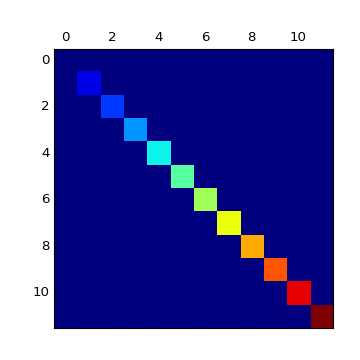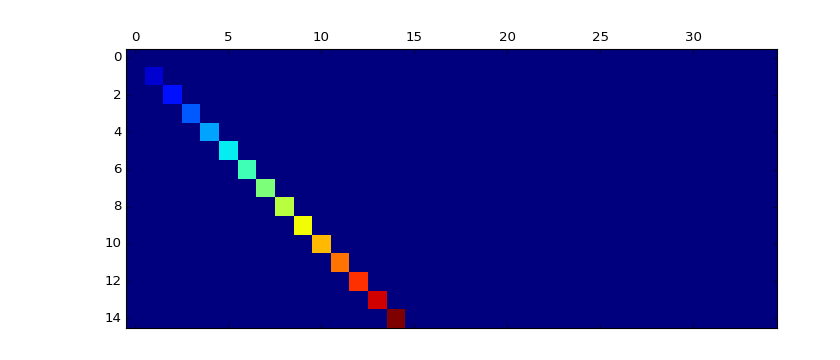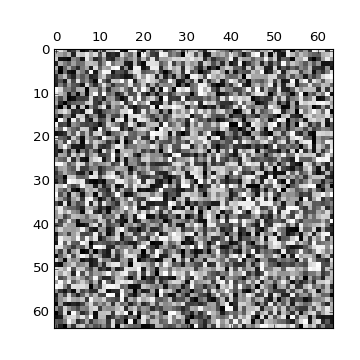### We're updating the default styles for Matplotlib 2.0

Learn what to expect in the new updatesTravis-CI:# pylab_examples example code: matshow.py¶(png, hires.png, pdf)(png, hires.png, pdf)(png, hires.png, pdf)

```"""Simple matshow() example."""
import matplotlib.pyplot as plt
import numpy as np

def samplemat(dims):
"""Make a matrix with all zeros and increasing elements on the diagonal"""
aa = np.zeros(dims)
for i in range(min(dims)):
aa[i, i] = i
return aa

# Display 2 matrices of different sizes
dimlist = [(12, 12), (15, 35)]
for d in dimlist:
plt.matshow(samplemat(d))

# Display a random matrix with a specified figure number and a grayscale
# colormap
plt.matshow(np.random.rand(64, 64), fignum=100, cmap=plt.cm.gray)

plt.show()
```

Keywords: python, matplotlib, pylab, example, codex (see Search examples)